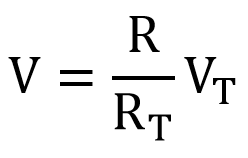## DC Series Circuits

A circuit is said to be a series circuit when several components are connected end to end paving only one way for the current to flow and the same current flows through each component. A series circuit is shown in the figure below.

FIGURE

In the series circuit, the current (I) is the same for all the components in the circuit. The voltage drop (VS) across each component is equal to the total supply voltage.

VS = V1 + V2 + V3

And the total resistance (RT) of the circuit is the sum of the individual resistors.

RT = R1 + R2 + R3

### Voltage Drop

As the electrons in the DC series circuit flow only one direction, one pole is always positive and the other pole is always positive and this is termed as polarity. This polarity is determined by the direction of conventional current from a positive to a more negative end. If we see the circuit in the following figure, the terminal of R1 connected to a point ‘A’ has a more positive potential than point ‘B’. Thus, point ‘A’ is more positive than point ‘B’, while point ‘D’ is more negative than point ‘C’.

FIGURE

When current flows through the resistor, a drop in voltage occurs. So, in order to give a division of voltage in a circuit, several resistors can be used in series across an applied voltage. The voltage difference between any two points in the circuit is known as potential difference and the resistors used are known as potential dividers.

Each resistance in a series circuit provides a voltage drop, which is equal to its proportional part of the applied voltage. The equation form is given below.V = voltage (V)

R = resistance (Ω)

RT = total resistance (Ω)

R/RT = proportional part of resistance (Ω)

VT = total voltage (V)